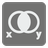# Boolean Algebra

Simple
DE EL English ES FR IT JA RU UK

In mathematics and mathematical logic, Boolean algebra is a sub-area of algebra in which the values of the variables are true or false, typically denoted with 1 or 0 respectively. Instead of elementary algebra where the values of the variables are numbers and the main operations are addition and multiplication, the main operations of Boolean algebra are the conjunction (denoted ∧), the disjunction (denoted ∨) and the negation (denoted ¬).

In this mission you should implement some boolean operations:
- "conjunction" denoted x ∧ y, satisfies x ∧ y = 1 if x = y = 1 and x ∧ y = 0 otherwise.
- "disjunction" denoted x ∨ y, satisfies x ∨ y = 0 if x = y = 0 and x ∨ y = 1 otherwise.
- "implication" (material implication) denoted x→y and can be described as ¬ x ∨ y. If x is true then the value of x → y is taken to be that of y. But if x is false then the value of y can be ignored; however the operation must return some truth value and there are only two choices, so the return value is the one that entails less, namely true.
- "exclusive" (exclusive or) denoted x ⊕ y and can be described as (x ∨ y)∧ ¬ (x ∧ y). It excludes the possibility of both x and y. Defined in terms of arithmetic it is addition mod 2 where 1 + 1 = 0.
- "equivalence" denoted x ≡ y and can be described as ¬ (x ⊕ y). It's true just when x and y have the same value.

Here you can see the truth table for these operations:

``` x | y | x∧y | x∨y | x→y | x⊕y | x≡y |
--------------------------------------
0 | 0 |  0  |  0  |  1  |  0  |  1  |
1 | 0 |  0  |  1  |  0  |  1  |  0  |
0 | 1 |  0  |  1  |  1  |  1  |  0  |
1 | 1 |  1  |  1  |  1  |  0  |  1  |
--------------------------------------
```

You are given two boolean values x and y as 1 or 0 and you are given an operation name as described earlier. You should calculate the value and return it as 1 or 0.

Input: Three arguments. X and Y as 0 or 1. An operation name as a string.

Output: The result as 1 or 0.

Example:

`assert boolean(0, 0, "conjunction") ==...`
You should be an authorized user in order to see the full description and start solving this mission.45
Settings
Code:
Other:
Invalid hot key. Each hot key should be unique and valid
Hot keys:
•  to Run Code: to Check Solution: to Stop:
CheckiO Extensions

CheckiO Extensions allow you to use local files to solve missions. More info in a blog post.

In order to install CheckiO client you'll need installed Python (version at least 3.8)

Install CheckiO Client first:

`pip3 install checkio_client`

`checkio --domain=py config --key=`

Sync solutions into your local folder

`checkio sync`

(in beta testing) Launch local server so your browser can use it and sync solution between local file end extension on the fly. (doesn't work for safari)

`checkio serv -d`

Alternatevly, you can install Chrome extension or FF addon

`checkio install-plugin`
`checkio install-plugin --ff`
`checkio install-plugin --chromium`

Read more here about other functionality that the checkio client provides. Feel free to submit an issue in case of any difficulties.